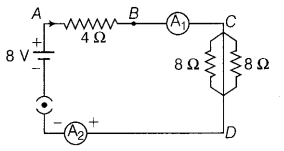# Find out the following in the electric circuit given in figure

Find out the following in the electric circuit given in figure.
(i) Effective resistance of two 8 \Omega resistors in the combination
(h) Current flowing through 4 \Omega resistor
(iii) Potential difference acorss 4 \Omega resistance
(iv) Power dissipated in 4 \Omega resistor
(v) Difference in ammeter readings if any1 Like